Preprocessing Window# Preprocessing Window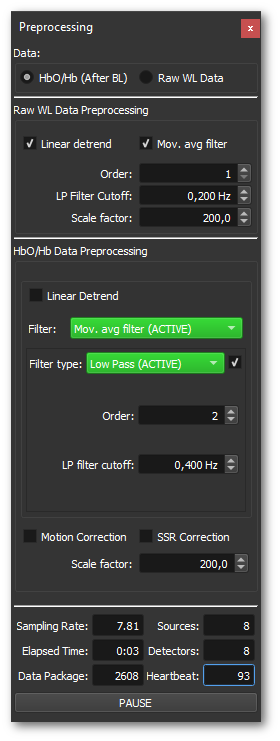The preprocessing window contains processing options of raw WL as well as HbO/Hb data. In the top of the window, the data (raw WL or HbO/Hb converted) that should be displayed in the plots can be selected.

The Raw WL data preprocessing options change the preprocessing of the raw WL data. For raw WL data, linear detrending and a moving average filter are available. The settings Order and LP Filter Cutoff change the moving average filter. The linear detrending option allows to remove linear trends from the raw WL data. This incremental procedure needs some time to settle down and produce stable results. The order defines how often the moving average filter should be applied. This allows for stronger filters, but also introduces a delay in the signal. Therefore, the decision of the filter order should be reasonable. The scale factor allows the modification of the scaling of the raw data.

In the HbO/Hb data processing section one can select the linear detrending and moving average option as in the raw WL preprocessing, but also apply different additional IIR filters:

Exponential moving average (EMA), Butterworth, RBJ Biquad, Chebyshev I, Chebyshev II, Elliptic, Bessel and Legendre.

To enable a specific filter, just choose a filter from the Filter: drop-down menu and check the Filter type: shown below. The drop-down menu of the filter types can contain different filter types for the selected filter above. To activate the filter, check the type using the little check box right to the filter type drop-down menu. The settings of the filter type are defined below the drop-down menu. Multiple filter types can be applied at the same time and are marked in green. It is also possible to mix filter types from different filters. Note that the filters are marked in green if one of the filter types of the individual filter is activated.

Note: For the moving average and exponential moving average filter the Order defines how often the specific filter should be applied. This can be useful if, for example, the heartbeat of a channel cannot be removed in only one go. Reapplying the filter using a higher order can solve this problem. Keep in mind that all these filters might introduce a time shift and that the strength of the shift depends on the selected parameters.

Some of the filters make use of the DSPFilter library:

“A Collection of Useful C++ Classes for Digital Signal Processing”
By Vincent Falco
Official project location:
——————————————————————————–
Copyright (c) 2009 by Vincent Falco

## Motion correction

The implemented motion correction is based on Correlation Based Signal Improvement (CBSI) Cui et. al., Functional Near Infrared Spectroscopy (NIRS) signal improvement based on negative correlation between oxygenated and deoxygenated hemoglobin dynamics, Neuroimage (2010). To turn on the motion correction, just check the “Motion Correction” check box. The main assumption consists of basically three components, true fNIRS signal, specific noise, which has similar effects on HbO and Hb (like noise induced by head motion) and is subject to constant factor, and white noise. F can be seen as external and it can be assumed that it is independent from the true fNIRS signal (xo and y0).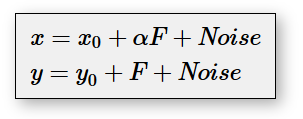As shown in the equation above, were x and y are the measured HbO and Hb signals, x0 and y0 are the true HBO and Hb values, and F is noise with an identical effect on both, HbO and Hb (which is subject to a positive constant factor a). The noise part consists of high frequency white noise introduced by the used NIRS hardware. This noise is not identical on HbO and Hb and therefore should be removed using standard filtering options. Therefore, the motion correction procedure is applied after filtering the data. To be able to determine x0 and y0, two assumptions are necessary:

1. x0 and y0 are negatively correlated
2. x0 and F are not or minimally correlated

The first assumption results in the following equation: x0 = −βy0. The free parameter β is accounting for the difference in amplitude of the HbO and Hb signal. Adding this assumption to the equation above results in the following equation: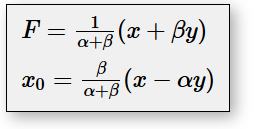When adding the assumption that α=β, as described by Cui and colleagues, α becomes: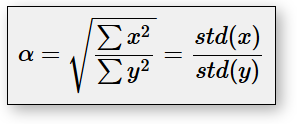This can now be easily inserted into the first equations, resulting in the final model for the CBSI motion correction: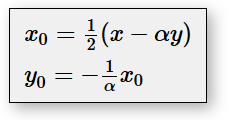The correction is performed, as stated above, before the SSR correction and after filtering. It is advised to filter the data in advance, when using this method, to fulfill the assumptions of the method. The correction is only applied on the preprocessed data as described before. In case no filtering is applied, the correction is applied on the raw HbO Hb signal.

## (S)hort (S)eparation (R)egression Correction

Short-distance measurements can be used to remove signal from extra-cerebral layers of the head, e.g., caused by blood flow changes. These signals can be recorded using the short-distance channels (0.5-1.0cm source detector separation distance) and removed from the standard fNIRS channels (~3.0cm source detector distance). To remove this signal, a method from Goodwin and colleagues is implemented in TSI:

J. R. Goodwin et. al., Short-channel functional near-infrared spectroscopy regressions improve when source- detector separation is reduced. Neurophotonics (2014).

The following equations are used to regress out the short-distance measurements from the respective long channel: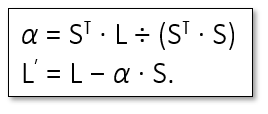S is the short-distance channel and L the long-distance (normal) channel; both are represented as column vectors. The SSR correction is performed as a last step in the preprocessing pipeline and is therefore applied in the fully preprocessed state for both, short-distance and long-distance channels. The SSR method described above is using only the closest short channel to correct the long distance channel, as it was shown to give best performance, see Gagnon et.al., Neuroimage 2012. The nearest short-distance channel is determined by the assumption that the short-distance detector is attached to each long-channel source in the NIRx cap setup. Since the method is performed in real-time a period of 120 frames is used in the beginning of the run to ensure a stable alpha.

To enable SSR just check the “SSR Correction” check box.

## Preprocessing Order

The preprocessing is applied in the following order:

1. Linear Detrend
2. Filtering (Filters and filter types are applied in same the order as they show up in the drop-down menu)
3. Motion Correction
4. SSR Correction

If a preprocessing option is skipped, the next following preprocessing step will be applied.

As for the raw WL options, also here the scale factor allows to modify the scaling of the HbO/Hb data.

At the bottom of the window, one can find status information of the currently running experiment. The pause button allows to pause and continue the processing.

More details about the processing in Turbo-Satori can be found in the corresponding article:
Lührs, M. and Goebel, R. (2019) ‘Turbo-Satori: a neurofeedback and brain–computer interface toolbox for real-time functional near-infrared spectroscopy’, Neurophotonics, 4(4), p. 1. doi: 10.1117/1.NPh.4.4.041504.